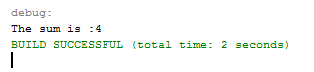# Sum Two Integers Value using Java

In my last tutorial entitle “Printing Hello Word in Java”, discuss the structure of Java programs and its parts. This tutorial will teach you on how you can sum two integers values using Java. Integer is one of the examples of Data type in Java.

Remember that there are many Data type that handles Number values and Integer can only handle whole numbers.

### Sum Two Integer Value in Java steps

1.Create a new project in Netbeans and name the project on what you want to name it.2.Insert a main method inside your Class.

[java]public static void main(String args[]){

}[/java]

3.Declare three integer variables and initialized it inside your new created main method.

[java]int num1=2;
int num2=2;
int total=0;[/java]

3.Insert the code below to calculate and display the result.

[java]total = num1 + num2;
System.out.println(“The sum is :” + total);[/java]

4. Run your program and the result should look like the image below.5. Complete source code

[java]public class SumTwoIntegers {
public static void main(String args[]){
int num1=2;
int num2=2;
int total=0;
total = num1 + num2;
System.out.println(“The sum is :” + total);
}
}[/java]

## If you have any comments or suggestion about on Sum Two Integers Value using Java, Please feel free to leave your comment below, use the contact page of this website or use my contact information.

Email: [email protected]  |  Cell. No.: 09468362375